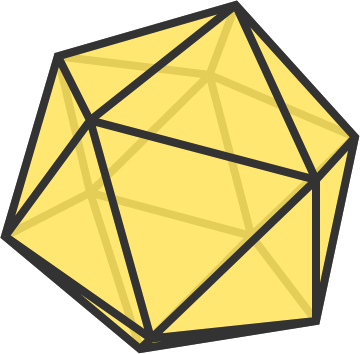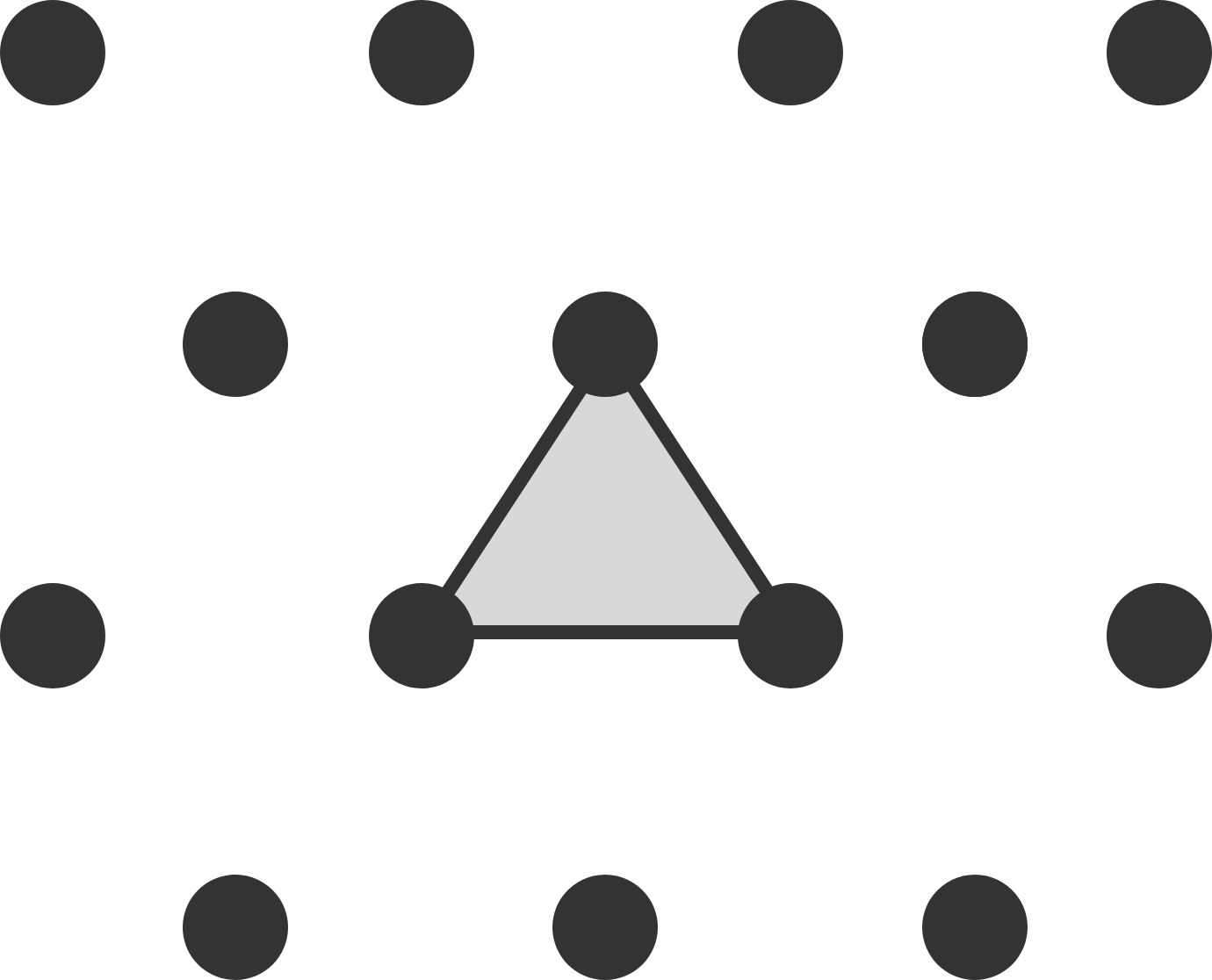# Problems of the Week

Contribute a problemAn icosahedron is rotated several times about different axes passing through its center.

Is it possible that none of the points on the surface of the icosahedron are in the same place as they were from before?

Note: The surface is considered the union of all faces, edges and vertices.

Pick’s theorem states that, given a polygon with vertices on a unit square lattice, the relationship between the area $A_S$ of the polygon, the number of boundary points $B$ of the polygon, and the number of interior points $I$ of the polygon is $A_S = I + \frac{1}{2}B - 1$.

There exists a similar relationship for a polygon with vertices on an equilateral triangle lattice in which the area of the smallest possible triangle is 1.The area of the shaded triangle is 1.

If $A_T$ is the area of the polygon, $B$ is the number of boundary points of the polygon, and $I$ is the number of interior points of the polygon, then the relationship is $A_T = aI + bB + c.$

What is $|a|+|b|+|c|?$

$\large \int_0^\infty\frac{\ln\frac{1+x^{11}}{1+x^3}}{(1+x^2)\ln x}\, \mathrm dx = \, ?$

The integral above has a closed form. Evaluate this integral and give your answer to three decimal places.A magician is so good at shuffling that his shuffles are always perfect in-shuffles. That means his shuffles are done in the following two steps:

1. Split the pile of cards exactly in half.
2. Interleave the top half with the bottom half such that every second card is from the top half.

If he started with six cards and shuffled thrice, he would get the original arrangement back.If he started with a deck of 52 cards, how many shuffles would he need to get the original arrangement back?

Let $\{a_0, a_1, a_2 \ldots, a_n\}$ be the set of integers that satisfy $\sum_{k=0}^{n} \binom{n}{k}a_k = F_n\ \ \forall n \in \{1,2,3,4,\ldots \},$ where $F_n$ is the $n^\text{th}$ Fibonacci number $(F_1=1, F_2=1, F_3=2, \ldots, F_{n+1} = F_n + F_{n-1}).$ Then we have $\lim_{n\rightarrow\infty} \frac{a_{n+1}}{a_n} = \frac{a+\sqrt{b}}{c},$ where $a$, $b$, and $c$ are integers with $a>0$ and $b$ square-free. What is $a+b+c?$

×